𓂀
Code
𓆖
Archaeological Site Map
𓁨
Tutankhamun's Papyrus
𓁢
Help
 1 2 3 4 5 6 7 8 9 10 11 12
```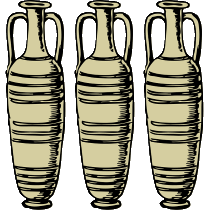amphora_A = 8
amphora_B = 6
amphora_A = amphora_A + amphora_B
amphora_C = amphora_A + amphora_B

key = amphora_C / 2

IF amphora_A < amphora_B THEN
key = key + 50
ELIF amphora_A > amphora_B THEN
key = key * 6
ELSE
key = key - 10
END IF

OUTPUT key```
```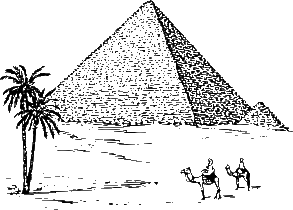#The Great Pyramid of Giza has 205 steps
steps = 205

FOR i FROM 1 to 20
steps = steps - 10
NEXT i

key = steps ^ 2

OUTPUT key

/* ----------------------------------
Help:
^ is the exponentiation operator:
a^b means a to the power of b.
-----------------------------------*/```
```#The Nile is 6,650 km long
#The Amazon is 6,400km long
nile = 6650
amazon = 6400

IF nile >= amazon THEN
key = (nile - amazon) / 2
ELSE:
key = (amazon - nile) / 5
END IF

OUTPUT key```
```key = 30

FOR i FROM 1 TO 5
key = key + i
NEXT i

OUTPUT key
```
```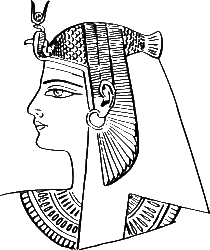key = 20

FOR i FROM 1 TO 6
FOR j FROM 1 TO 7
key = key + 1
NEXT j
NEXT i

OUTPUT Key```
```FUNCTION getVolumePyramid(width,length,height)
base = width * length
volume = base * height / 3
return volume
END FUNCTION

w = 6
l = 6
h = 3
key = getVolumePyramid(w, l, h)

OUTPUT key```
```FUNCTION isEven(number)
IF number MOD 2 == 0 THEN
RETURN True
ELSE
RETURN False
END IF
END FUNCTION

list = [4,8,10,7,4,5,6,18]
i = 0
key = list

WHILE isEven(key) == True
i = i + 1
key = list[i]
END WHILE

key = key * i

OUTPUT key
```
```key = 3
WHILE key < 50
key = key * 2
IF key >20 AND key<=24 THEN
key = key - 3
END IF
END WHILE

OUTPUT key```
```#GPS Coordinates of the Great Sphinx of Giza
latitude = 29.9718
longitude = 31.1360
key = ROUND(longitude,0)

IF latitude>30 THEN
IF longitude>30 THEN
key = key * 3
ELSE
key = key + 30
END IF
ELSE
IF longitude>30 THEN
key = key + 20
ELSE
key = key * 2
END IF
END IF
OUTPUT key```
```high = 14
low = 4

WHILE high > low
low = low + 1
high = high -1
END WHILE

key = high ^ 2

OUTPUT key```
```queen = "NEFERTITI"
character = RIGHT(queen,1)
key = ASCII(character)

quotient = key DIV 10
remainder = key MOD 10

key = (quotient * remainder) + 2

OUTPUT key

/* --------------------------------------
Help:
DIV is the quotient of a whole division,
MOD is the remainder of a whole division.
-----------------------------------------*/```
```queen = "CLEOPATRA"

first = LEFT(queen,1)
last = RIGHT(queen,1)

key1 = ASCII(first)
key2 = ASCII(last)
key3 = LENGTH(queen)
key = key1 + key2 + key3

OUTPUT Key

/* HELP: The ASCII code for character A is 65 */```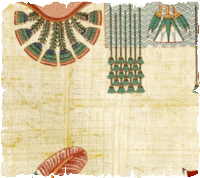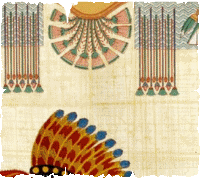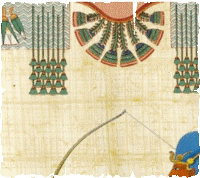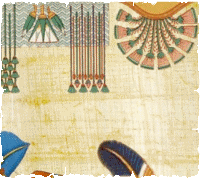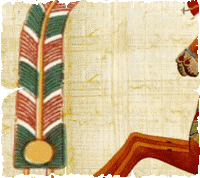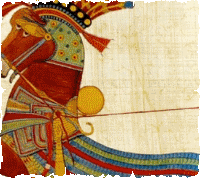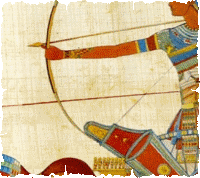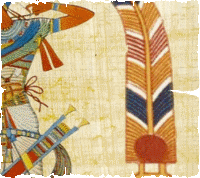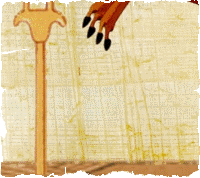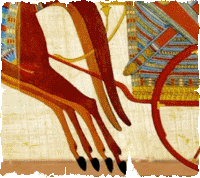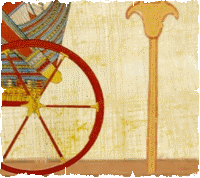There is nothing here!
Please carry on with your archaeological excavations!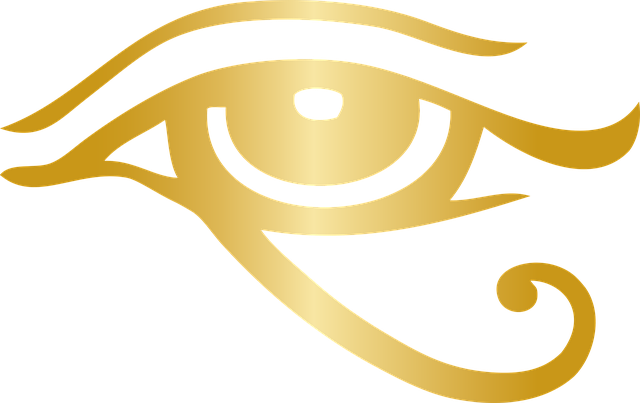You have found one piece of Tutankhamun's papyrus:```String Manipulation
string = "abcdef"
LENGTH(string) would return the number of characters in the string (e.g. 6)
LEFT(string,3) would return the first 3 characters of the string (e.g. "abc")
RIGHT(string,3) would return the last 3 characters of the string (e.g. "def")

MOD & DIV
DIV is the quotient of a division, whereas MOD is the remainder.
For instance: 20 / 3 = 6 remainder 2
So:
20 DIV 3 would return 6
20 MOD 3 would return 2

ASCII Codes
Check the following ASCII table to lookup the right codes.
```